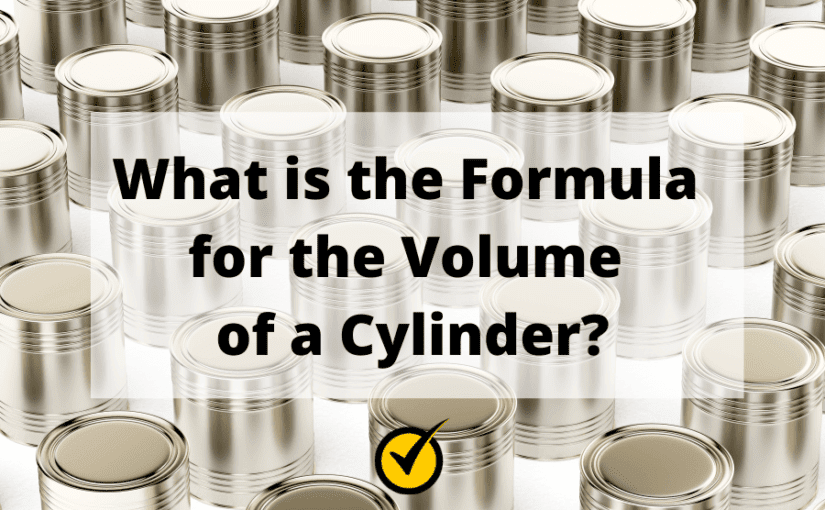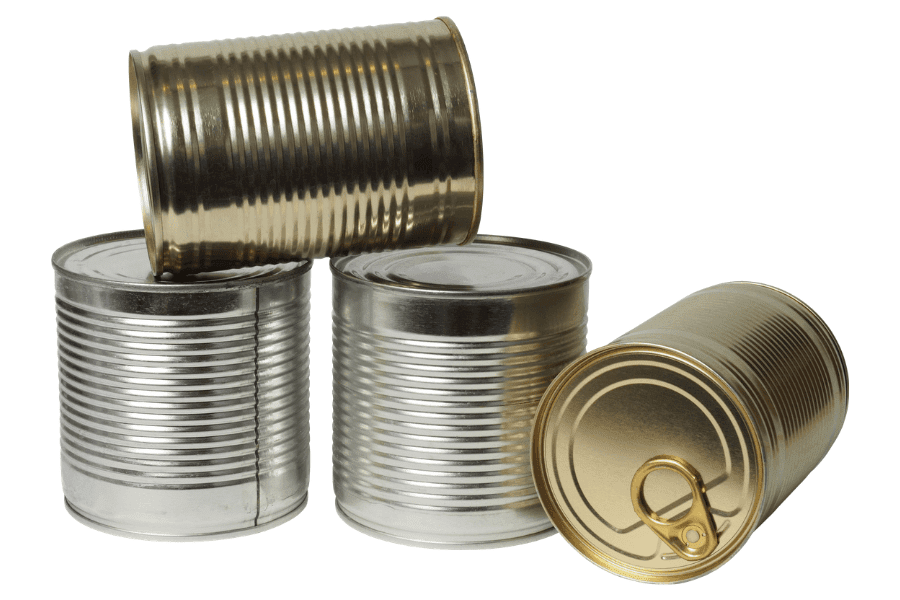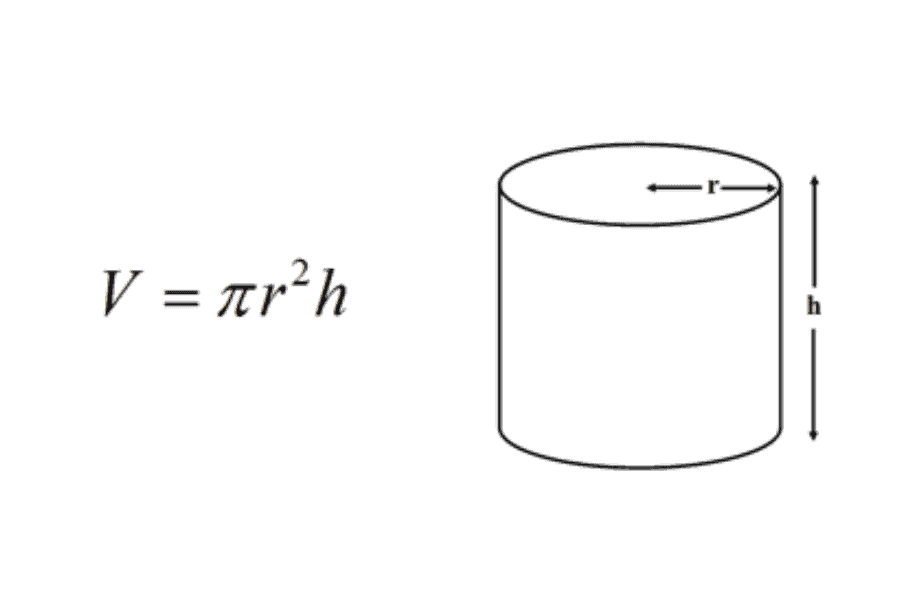# What is the Formula for the Volume of a Cylinder?

A cylinder is just one of the basic shapes you’ll learn in math class.

A cylinder is a three-dimensional shape, like a can of soda or a can of soup. It has two round flat bases (the top and bottom) that are of equal size and a curved side. The two flat bases are connected by the curved side that makes the shape look like a tube.

The volume of a cylinder tells you how much space the cylinder has on the inside such as to fill up with soup.To be able to find out how much soup can fill the can, you’ll need to know the height and the radius of the cylinder. The height is the distance between the top and the bottom bases; how tall the can is. The radius is half of the length of the top and bottom.

Once you find the height and radius of the cylinder, you’ll be able to find the volume.

To find the volume of a cylinder, you will use this formula: